##Fred's ImageMagick Scripts

 Licensing: Copyright © Fred Weinhaus My scripts are available free of charge for non-commercial (non-profit) use, ONLY. For use of my scripts in commercial (for-profit) environments or non-free applications, please contact me (Fred Weinhaus) for licensing arrangements. My email address is fmw at alink dot net. If you: 1) redistribute, 2) incorporate any of these scripts into other free applications or 3) reprogram them in another scripting language, then you must contact me for permission, especially if the result might be used in a commercial or for-profit environment. Usage, whether stated or not in the script, is restricted to the above licensing arrangements. It is also subject, in a subordinate manner, to the ImageMagick license, which can be found at: http://www.imagemagick.org/script/license.php Please read the Pointers For Use on my home page to properly install and customize my scripts.

## OMNISTRETCH

 Enhances the dynamic range of brightness values and optionally saturation values in an image

Download Script

last modified: December 15, 2018

 USAGE: omnistretch [option1] [option2] [option3] [option4] infile outfile Option1 (choice of one only): -h or -help ................ get help -st ........................ get image statistics; requires input_file -m or -model ............... processing (color) model (HSL, HSB, RGB); ............................ requires infile and outfile; default HSL if none specified Option2 (choice of one only): -b ... low,[high[,gamma]] .. process w/ brightness 0-100 for low,high; gamma 0-10 -c ... low,[high[,gamma]] .. process w/ histogram counts >=0 for low,high; gamma 0-10 -pc .. low,[high[,gamma]] .. process w/ % of max histogram counts 0-100 for low,high; gamma 0-10 -cc .. low,[high[,gamma]] .. process w/ cumulative histogram counts >=0 for low,high; gamma 0-10 -cd .. low,[high[,gamma]] .. process w/ cumulative histogram count differences >=0 for low,high; gamma 0-10 -ab .. gamma_method ........ auto process w/ default brightness values of min,max,gamma -ac .. gamma_method ........ auto process w/ default histogram counts of 30,30,gamma -apc . gamma_method ........ auto process w/ default % of max histogram counts of 10,10,gamma -acc . gamma_method ........ auto process w/ default % cumulative histogram counts of .6,.6,gamma -acd . gamma_method ........ auto process w/ default % cumulative histogram count differences of .06,.06,gamma Option2: -s ... sgamma .............. process w/ saturation; sgamma 0-10 Option3: -g ......................... display graph of intensity mapping function, ............................ but must be used in conjunction with option 1 or 2, ............................ but not -h or -help or -st PURPOSE: To enhance the dynamic range of brightness values and optionally saturation values in an image. DESCRIPTION: OMNISTRETCH is designed to enhance the dynamic range of brightness values and optionally saturation values in an image. It has various means of linearly doing this as described below. If a gamma value is also supplied, then a non-linear change is affected on top of the linear change. It works by computing a grayscale histogram, which is then used to compute a low and high value to map to black and white, respectively in the image as a whole or on either the Lightness or Brightness channels, depending upon which colorspace model is selected. The script uses the IM -level function to do the processing. If a saturation change is desired, then the IM -gamma function will be applied to the saturation channel. No saturation processing is allowed if the image is grayscale. The graph is normally just viewed, but a default parameter in the program can be set to allow it to be saved as outfilename_graph.gif. To end the script, close/quit the graph image. Argument: ........ displays help information. Argument: -h ..... displays help information. Argument: -g ..... displays graph of intensity mapping function. Argument: -st .... displays image statistics, including: minimum, maximum, mean, standard deviation and histogram in a range of brightness values scaled to 0-100. An input_file is required with no output_file specified. Argument: -m --- defines the processing color model to use for the processing of a color image. If HSL or HSB are specified, then the the image is first transformed into that color space and the corresponding channels are separated. Either the L or B channels will then be used to compute a histogram, which is then used to determine the low and high values to map to black and white, within that channel. The channels are then put back together and output in RGB format. If RGB is selected, the image is first converted to RGB format and used to generate a grayscale image. The grayscale image is then used to compute a histogram. The histogram is then used to determine the low and high values which are then stretched in the RGB image to black and white, respectively. The default for a color image is HSL. If a grayscale image is the input file, then the processing color model is ignored. Note that results for HSB tend to be undersaturated and for RGB tend to be oversaturated. Argument: -b ..... defines the method using brightness values for low and high in the range of 0-100 to be linearly stretch to full black (0) and full white (100). If high is not provided, high=100-low. A value of 0,[100,] leaves the image unchanged. Optionally, a gamma value may be included in the range of 0 upward; but, nominally 0-10, where 1 is no change, <1 is less contrast and >1 is more contrast. Gamma is a non-linear adjustment. Argument: -c ..... defines the method using histogram counts for low and high. The method finds the first occurrences of the specified count (or higher) from each end of the histogram to locate the corresponding low and high brightness values to linearly stretch to full black (0) and full white (100). If only one count is specified, it will be used for both low and high. A value of 0,[0,] leaves the image unchanged. Optionally, a gamma value may be included in the range of >=0; but, nominally 0-10, where 1 (default) is no change, <1 is less contrast and >1 is more contrast. Gamma is a non-linear adjustment. Argument: -pc .... defines the method using percent of maximum histogram counts for low and high. The method finds the first occurrences of the specified percent of maximum histogram count (or higher) from each end of the histogram to locate the corresponding low and high brightness values to linearly stretch to full black (0) and full white (100). If only one count is specified, it will be used for both low and high. Counts for low and high are expressed as percent of the maximum count in the histogram, but the % symbol is not specified. A value of 0,[0,] leaves the image unchanged. Optionally, a gamma value may be included in the range of >=0; but, nominally 0-10, where 1 (default) is no change, <1 is less contrast and >1 is more contrast. Gamma is a non-linear adjustment. Argument: -cc .... defines the method using cumulative histogram counts in percent of total count for low and high. The method finds the first occurrences of the specified cumulative count (or higher) from each end of the histogram to locate the corresponding low and high brightness values to linearly stretch to full black (0) and full white (100). If only one cumulative count is specified, it will be used for both low and high. A value of 0,[0,] indicates no change in the image. Cumulative counts for low and high are expressed as percent of total pixels in the image, but the % symbol is not specified. Optionally, a gamma value may be included in the range of >=0; but, nominally 0-10, where 1 (default) is no change, <1 is less saturation and >1 is more saturation. Gamma is a non-linear adjustment. Argument: -cd .... defines the method using cumulative percent histogram count differences between successive histogram values equal to or exceeding low and high. The method finds the first occurrences of the specified cumulative count differences (or higher) from each end of the histogram to locate the corresponding low and high brightness values to linearly stretch to full black (0) and full white (100). If only one cumulative count difference is specified, it will be used for both low and high. A value of 0,[0,] indicates no change in the image. Cumulative count differences for low and high are expressed as percent of total pixels in the image, but the % symbol is not specified. This method is basically looking for where the slope of the histogram, starting from each end, increases above a threshold given by low and high. Optionally, a gamma value may be included in the range of >=0; but, nominally 0-10, where 1 (default) is no change, <1 is less saturation and >1 is more saturation. Gamma is a non-linear adjustment. Argument: -ab .... automatic version of -b using the image's actual minimum and maximum values for low and high. gamma_method=1 sets gamma=1. gamma_method=2 determines gamma from the computed low and high. gamma_method=3 determines gamma from the image's standard deviation. Argument: -ac .... automatic version of -c using low and high counts of 30. gamma_method=1 sets gamma=1. gamma_method=2 determines gamma from computed low and high. gamma_method=3 determines gamma from the image's standard deviation. Argument: -apc ... automatic version of -pc using low and high percent of maximum counts of 10. gamma_method=1 sets gamma=1. gamma_method=2 determines gamma from computed low and high. gamma_method=3 determines gamma from the image's standard deviation. Argument: -acc ... automatic version of -cc using low and high cumulative counts of 0.6. gamma_method=1 sets gamma=1. gamma_method=2 determines gamma from computed low and high. gamma_method=3 determines gamma from the image's standard deviation Argument: -acd ... automatic version of -cd using low and high cumulative count differences of 0.06. gamma_method=1 sets gamma=1. gamma_method=2 determines gamma from computed low and high. gamma_method=3 determines gamma from the image's standard deviation OBSERVATION: All these algorithms tend to work better on image's which have long, low histogram tails or do not span the full range of 0-100. Typically, this means low contrast images. REMARK: Feel free to change the default values below for the automatic methods: -ac, -apc and -acc, if you find that those values work better for your class of imagery. Also the methods for automatically determining gamma are not well tested. Method 2 is limited to histograms for which low > 0 and/or high < 100. Method 3 seems to work for both low and high contrast images. CAVEAT: No guarantee that this script will work on all platforms, nor that trapping of inconsistent parameters is complete and foolproof. Use At Your Own Risk.

### EXAMPLES

Autolevels

 Original Image artificially brightened and contrast lowered convert lena.jpg -fx ".724u+.310" lenap.jpghistogram min and max graylevels are automatically stretched to 0 and 100 (0 and 255) Histogram Grayscale Value Mapped To Black = 50.5 (128 in range 0 - 255) Histogram Grayscale Value Mapped To White = 100.0 (255 in range 0 - 255) Break Points = 0,0 50.5,0 100.0,100.0 100.0,100.0 arguments: -m HSB -ab 1 -g arguments: -m HSB -ab 1 -s 1.5same but with saturation increased by 50%; graph will be the same and does not show such changes

 The example above on the right does the following: Separates the RGB image into HSB channels. Applies the linear stretch to the Brightness channel from graylevel 50.5 to black and 100 to white Applies a gamma of 1.5 to the Saturation channel. Merges the Hue, Saturation and Brightness channels back together as an RGB image. This is equivalent to the following IM commands: convert lenap.jpg -colorspace HSB -channel R -separate tmp0.jpg convert lenap.jpg -colorspace HSB -channel G -separate tmp1.jpg convert lenap.jpg -colorspace HSB -channel B -separate tmp2.jpg convert tmp2.jpg -level 50.5%,100.0%,1 tmp2p.jpg convert tmp1.jpg -gamma 1.5 tmp1p.jpg convert tmp0.jpg -colorspace HSB tmp0.jpg -compose CopyRed -composite \ tmp1p.jpg -compose CopyGreen -composite tmp2p.jpg -compose CopyBlue -composite \ -colorspace RGB lenap-hsbmethod-ab1_s1p5.jpg

 Autolevels: -ab and Processing Model: HSL first occurrence of graylevel from either end corresponding to cumulative histogram count=0.6% are automatically stretched to 0 and 100 (0 and 255) Histogram Grayscale Value Mapped To Black = 41.7 (106 in range 0 - 255) Histogram Grayscale Value Mapped To White = 96.2 (245 in range 0 - 255) Break Points = 0,0 41.7,0 96.2,100.0 100.0,100.0 source image arguments: -m HSL -ab 1 -g arguments: -m HSL -ab 2 -g arguments: -m HSL -ab 3 -g original image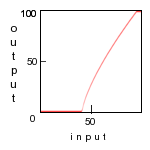Autolevels: -ab and Processing Model: HSB first occurrence of graylevel from either end corresponding to cumulative histogram count=0.6% are automatically stretched to 0 and 100 (0 and 255) Histogram Grayscale Value Mapped To Black = 50.5 (128 in range 0 - 255) Histogram Grayscale Value Mapped To White = 100.0 (255 in range 0 - 255) Break Points = 0,0 50.5,0 100.0,100.0 100.0,100.0 source image arguments: -m HSB -ab 1 -g arguments: -m HSB -ab 2 -g arguments: -m HSB -ab 3 -g original imageAutolevels: -ab and Processing Model: RGB first occurrence of graylevel from either end corresponding to cumulative histogram count=0.6% are automatically stretched to 0 and 100 (0 and 255) Histogram Grayscale Value Mapped To Black = 37.2 (94 in range 0 - 255) Histogram Grayscale Value Mapped To White = 92.5 (235 in range 0 - 255) Break Points = 0,0 37.2,0 92.5,100.0 100.0,100.0 source image arguments: -m RGB -ab 1 -g arguments: -m RGB -ab 2 -g arguments: -m RGB -ab 3 -g original image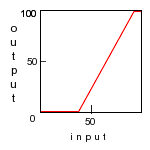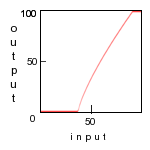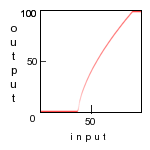Autolevels: -acc and Processing Model: HSL first occurrence of graylevel from either end corresponding to cumulative histogram count=0.6% are automatically stretched to 0 and 100 (0 and 255) Histogram Grayscale Value Mapped To Black = 44.7 (113 in range 0 - 255) Histogram Grayscale Value Mapped To White = 92.5 (235 in range 0 - 255) Break Points = 0,0 44.7,0 92.5,100.0 100.0,100.0 source image arguments: -m HSL -acc 1 -g arguments: -m HSL -acc 2 -g arguments: -m HSL -acc 3 -g original imageAutolevels: -acc and Processing Model: HSB first occurrence of graylevel from either end corresponding to cumulative histogram count=0.6% are automatically stretched to 0 and 100 (0 and 255) Histogram Grayscale Value Mapped To Black = 53.7 (136 in range 0 - 255) Histogram Grayscale Value Mapped To White = 100.0 (255 in range 0 - 255) Break Points = 0,0 53.7,0 100.0,100.0 100.0,100.0 source image arguments: -m HSB -acc 1 -g arguments: -m HSB -acc 2 -g arguments: -m HSB -acc 3 -g original image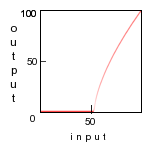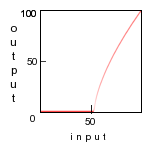Autolevels: -acc and Processing Model: RGB first occurrence of graylevel from either end corresponding to cumulative histogram count=0.6% are automatically stretched to 0 and 100 (0 and 255) Histogram Grayscale Value Mapped To Black = 43.9 (111 in range 0 - 255) Histogram Grayscale Value Mapped To White = 86.2 (219 in range 0 - 255) Break Points = 0,0 43.9,0 86.2,100.0 100.0,100.0 source image arguments: -m RGB -acc 1 -g arguments: -m RGB -acc 2 -g arguments: -m RGB -acc 3 -g original image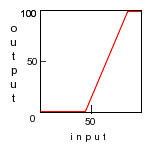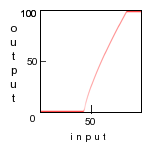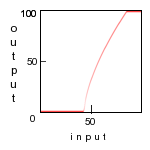The example above for arguments: -m HSB -acc 3 does the following: Separates the RGB image into HSB channels. Applies the linear stretch to the Brightness channel from graylevel 53.7 to black and 100 to white. and also applies a gamma of 1.52 to the Brightness channel. Merges the Hue, Saturation and Brightness channels back together as an RGB image. This is equivalent to the following IM commands: convert lenap.jpg -colorspace HSB -channel R -separate tmp0.jpg convert lenap.jpg -colorspace HSB -channel G -separate tmp1.jpg convert lenap.jpg -colorspace HSB -channel B -separate tmp2.jpg convert tmp2.jpg -level 53.7%,100.0%,1.52 tmp2p.jpg convert tmp0.jpg -colorspace HSB tmp0.jpg -compose CopyRed -composite \ tmp1.jpg -compose CopyGreen -composite tmp2p.jpg -compose CopyBlue -composite \ -colorspace RGB lenap-hsbmethod-acc3.jpg

Autolevels From Other Imaging Tools

 Photoshop GraphicConverter GIMPOption -st: Image Statistics (for artificially modified image and processing model HSB)

These are provided to assist in the manual selection of low and high values to map to black and white, respectively.

Image Statistics:

Colorspace = RGB

Width = 128
Height = 128
Total Pixels = 16384

Min (0-100) = 50.5
Max (0-100) = 100.0
Mean (0-100) = 82.2
Std (0-...) = 13.5

Histogram:

Total Values=127
Mid Point=63
Max Count = 511
Tot Count = 16384

B=brightness 0-100(0-255)
C=count
PC=percent of maximum count
CC=cumulative count percent
CD=cumulative count percent difference

 1 B=50.5(128) C=3 PC=.5 CC=.01 CD=.01 2 B=50.9(129) C=1 PC=.1 CC=.02 CD=0 3 B=51.3(130) C=7 PC=1.3 CC=.06 CD=.04 4 B=51.7(131) C=10 PC=1.9 CC=.12 CD=.06 5 B=52.1(132) C=12 PC=2.3 CC=.20 CD=.07 6 B=52.5(133) C=17 PC=3.3 CC=.30 CD=.10 7 B=52.9(134) C=15 PC=2.9 CC=.39 CD=.09 8 B=53.3(135) C=17 PC=3.3 CC=.50 CD=.10 9 B=53.7(136) C=27 PC=5.2 CC=.66 CD=.16 10 B=54.1(137) C=35 PC=6.8 CC=.87 CD=.21 11 B=54.5(138) C=42 PC=8.2 CC=1.13 CD=.25 12 B=54.9(139) C=63 PC=12.3 CC=1.51 CD=.38 13 B=55.2(140) C=67 PC=13.1 CC=1.92 CD=.40 14 B=55.6(141) C=87 PC=17.0 CC=2.45 CD=.53 15 B=56.0(142) C=108 PC=21.1 CC=3.11 CD=.65 16 B=56.4(143) C=115 PC=22.5 CC=3.82 CD=.70 17 B=56.8(144) C=132 PC=25.8 CC=4.62 CD=.80 18 B=57.2(145) C=148 PC=28.9 CC=5.52 CD=.90 19 B=57.6(146) C=138 PC=27.0 CC=6.37 CD=.84 20 B=58.0(147) C=168 PC=32.8 CC=7.39 CD=1.02 21 B=58.4(148) C=168 PC=32.8 CC=8.42 CD=1.02 22 B=58.8(149) C=169 PC=33.0 CC=9.45 CD=1.03 23 B=59.2(150) C=132 PC=25.8 CC=10.26 CD=.80 24 B=59.6(151) C=150 PC=29.3 CC=11.17 CD=.91 25 B=60.0(153) C=115 PC=22.5 CC=11.87 CD=.70 26 B=60.3(153) C=117 PC=22.8 CC=12.59 CD=.71 27 B=60.7(154) C=107 PC=20.9 CC=13.24 CD=.65 28 B=61.1(155) C=98 PC=19.1 CC=13.84 CD=.59 29 B=61.5(156) C=86 PC=16.8 CC=14.36 CD=.52 30 B=61.9(157) C=85 PC=16.6 CC=14.88 CD=.51 31 B=62.3(158) C=98 PC=19.1 CC=15.48 CD=.59 32 B=62.7(159) C=69 PC=13.5 CC=15.90 CD=.42 33 B=63.1(160) C=90 PC=17.6 CC=16.45 CD=.54 34 B=63.5(161) C=65 PC=12.7 CC=16.85 CD=.39 35 B=63.9(162) C=64 PC=12.5 CC=17.24 CD=.39 36 B=64.3(163) C=61 PC=11.9 CC=17.61 CD=.37 37 B=64.7(164) C=57 PC=11.1 CC=17.96 CD=.34 38 B=65.0(165) C=58 PC=11.3 CC=18.31 CD=.35 39 B=65.4(166) C=63 PC=12.3 CC=18.70 CD=.38 40 B=65.8(167) C=58 PC=11.3 CC=19.05 CD=.35 41 B=66.2(168) C=58 PC=11.3 CC=19.40 CD=.35 42 B=66.6(169) C=44 PC=8.6 CC=19.67 CD=.26 43 B=67.0(170) C=57 PC=11.1 CC=20.02 CD=.34 44 B=67.4(171) C=72 PC=14.0 CC=20.46 CD=.43 45 B=67.8(172) C=53 PC=10.3 CC=20.78 CD=.32 46 B=68.2(173) C=67 PC=13.1 CC=21.19 CD=.40 47 B=68.6(174) C=71 PC=13.8 CC=21.63 CD=.43 48 B=69.0(175) C=64 PC=12.5 CC=22.02 CD=.39 49 B=69.4(176) C=58 PC=11.3 CC=22.37 CD=.35 50 B=69.8(177) C=62 PC=12.1 CC=22.75 CD=.37 51 B=70.1(178) C=47 PC=9.1 CC=23.04 CD=.28 52 B=70.5(179) C=52 PC=10.1 CC=23.35 CD=.31 53 B=70.9(180) C=58 PC=11.3 CC=23.71 CD=.35 54 B=71.3(181) C=67 PC=13.1 CC=24.12 CD=.40 55 B=71.7(182) C=59 PC=11.5 CC=24.48 CD=.36 56 B=72.1(183) C=69 PC=13.5 CC=24.90 CD=.42 57 B=72.5(184) C=74 PC=14.4 CC=25.35 CD=.45 58 B=72.9(185) C=81 PC=15.8 CC=25.84 CD=.49 59 B=73.3(186) C=50 PC=9.7 CC=26.15 CD=.30 60 B=73.7(187) C=63 PC=12.3 CC=26.53 CD=.38 61 B=74.1(188) C=53 PC=10.3 CC=26.86 CD=.32 62 B=74.5(189) C=65 PC=12.7 CC=27.25 CD=.39 63 B=74.9(190) C=69 PC=13.5 CC=27.67 CD=.42 64 B=75.2(191) C=83 PC=16.2 CC=72.32 CD=.50 65 B=75.6(192) C=108 PC=21.1 CC=71.81 CD=.65 66 B=76.0(193) C=82 PC=16.0 CC=71.15 CD=.50 67 B=76.4(194) C=82 PC=16.0 CC=70.65 CD=.50 68 B=76.8(195) C=88 PC=17.2 CC=70.15 CD=.53 69 B=77.2(196) C=81 PC=15.8 CC=69.61 CD=.49 70 B=77.6(197) C=82 PC=16.0 CC=69.12 CD=.50 71 B=78.0(198) C=90 PC=17.6 CC=68.62 CD=.54 72 B=78.4(199) C=90 PC=17.6 CC=68.07 CD=.54 73 B=78.8(200) C=100 PC=19.5 CC=67.52 CD=.61 74 B=79.2(201) C=103 PC=20.1 CC=66.91 CD=.62 75 B=79.6(202) C=125 PC=24.4 CC=66.28 CD=.76 76 B=80.0(204) C=125 PC=24.4 CC=65.52 CD=.76 77 B=80.3(204) C=154 PC=30.1 CC=64.75 CD=.93 78 B=80.7(205) C=166 PC=32.4 CC=63.81 CD=1.01 79 B=81.1(206) C=187 PC=36.5 CC=62.80 CD=1.14 80 B=81.5(207) C=155 PC=30.3 CC=61.66 CD=.94 81 B=81.9(208) C=152 PC=29.7 CC=60.71 CD=.92 82 B=82.3(209) C=133 PC=26.0 CC=59.79 CD=.81 83 B=82.7(210) C=114 PC=22.3 CC=58.97 CD=.69 84 B=83.1(211) C=112 PC=21.9 CC=58.28 CD=.68 85 B=83.5(212) C=125 PC=24.4 CC=57.59 CD=.76 86 B=83.9(213) C=124 PC=24.2 CC=56.83 CD=.75 87 B=84.3(214) C=157 PC=30.7 CC=56.07 CD=.95 88 B=84.7(215) C=169 PC=33.0 CC=55.12 CD=1.03 89 B=85.0(216) C=164 PC=32.0 CC=54.08 CD=1.00 90 B=85.4(217) C=163 PC=31.8 CC=53.08 CD=.99 91 B=85.8(218) C=180 PC=35.2 CC=52.09 CD=1.09 92 B=86.2(219) C=187 PC=36.5 CC=50.99 CD=1.14 93 B=86.6(220) C=203 PC=39.7 CC=49.85 CD=1.23 94 B=87.0(221) C=172 PC=33.6 CC=48.61 CD=1.04 95 B=87.4(222) C=177 PC=34.6 CC=47.56 CD=1.08 96 B=87.8(223) C=192 PC=37.5 CC=46.48 CD=1.17 97 B=88.2(224) C=221 PC=43.2 CC=45.31 CD=1.34 98 B=88.6(225) C=252 PC=49.3 CC=43.96 CD=1.53 99 B=89.0(226) C=271 PC=53.0 CC=42.42 CD=1.65 100 B=89.4(227) C=285 PC=55.7 CC=40.77 CD=1.73 101 B=89.8(228) C=285 PC=55.7 CC=39.03 CD=1.73 102 B=90.1(229) C=276 PC=54.0 CC=37.29 CD=1.68 103 B=90.5(230) C=240 PC=46.9 CC=35.60 CD=1.46 104 B=90.9(231) C=239 PC=46.7 CC=34.14 CD=1.45 105 B=91.3(232) C=266 PC=52.0 CC=32.68 CD=1.62 106 B=91.7(233) C=227 PC=44.4 CC=31.06 CD=1.38 107 B=92.1(234) C=197 PC=38.5 CC=29.67 CD=1.20 108 B=92.5(235) C=196 PC=38.3 CC=28.47 CD=1.19 109 B=92.9(236) C=237 PC=46.3 CC=27.27 CD=1.44 110 B=93.3(237) C=254 PC=49.7 CC=25.83 CD=1.55 111 B=93.7(238) C=313 PC=61.2 CC=24.27 CD=1.91 112 B=94.1(239) C=336 PC=65.7 CC=22.36 CD=2.05 113 B=94.5(240) C=317 PC=62.0 CC=20.31 CD=1.93 114 B=94.9(241) C=306 PC=59.8 CC=18.38 CD=1.86 115 B=95.2(242) C=260 PC=50.8 CC=16.51 CD=1.58 116 B=95.6(243) C=230 PC=45.0 CC=14.92 CD=1.40 117 B=96.0(244) C=173 PC=33.8 CC=13.52 CD=1.05 118 B=96.4(245) C=164 PC=32.0 CC=12.46 CD=1.00 119 B=96.8(246) C=146 PC=28.5 CC=11.46 CD=.89 120 B=97.2(247) C=170 PC=33.2 CC=10.57 CD=1.03 121 B=97.6(248) C=181 PC=35.4 CC=9.53 CD=1.10 122 B=98.0(249) C=194 PC=37.9 CC=8.43 CD=1.18 123 B=98.4(250) C=186 PC=36.3 CC=7.25 CD=1.13 124 B=98.8(251) C=189 PC=36.9 CC=6.11 CD=1.15 125 B=99.2(252) C=168 PC=32.8 CC=4.96 CD=1.02 126 B=99.6(253) C=134 PC=26.2 CC=3.93 CD=.81 127 B=100.0(255) C=511 PC=100.0 CC=3.11 CD=3.11